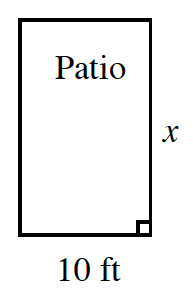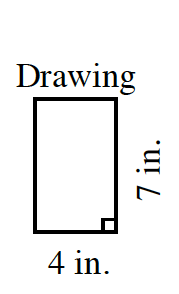### Home > CC2MN > Chapter 4 > Lesson 4.3.1 > Problem4-84

4-84.

Daniel needed to paint his patio, so he made a scale drawing of it. He knows the width of the patio is $10$ feet, but the scale drawing is in inches.

1. Find the length of the patio in feet.

Find the ratio between two corresponding sides and use it to create a proportion or to multiply as a scale factor and find the missing length.

$x=17.5$ feet

2. Find the area of the patio so Daniel knows how much paint to buy.

Use the dimensions of the patio to find the area by multiplying.

Patio Area: $175$ square feet

3. One can of paint covers $125$ square feet. How many cans of paint will Daniel need to buy?

If each can of paint covers $125$ square feet, how many cans are needed to cover $175$ square feet?

Daniel is only able to buy full cans of paint. Round up the cans of paint if it does not come out to an even full number.